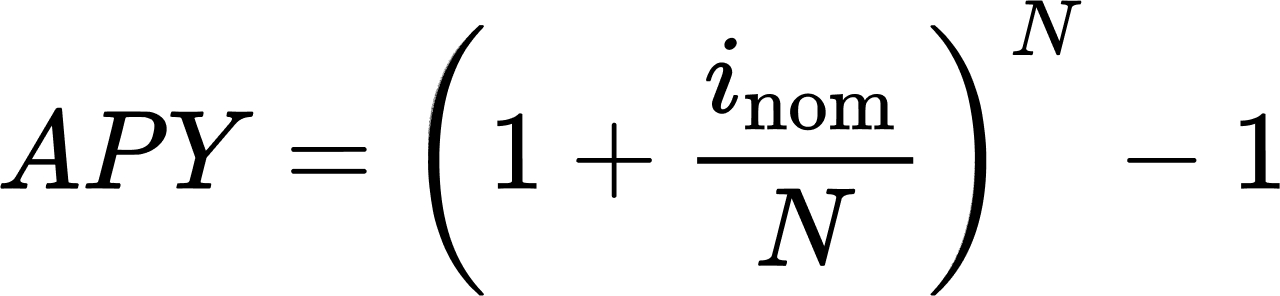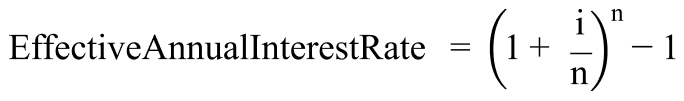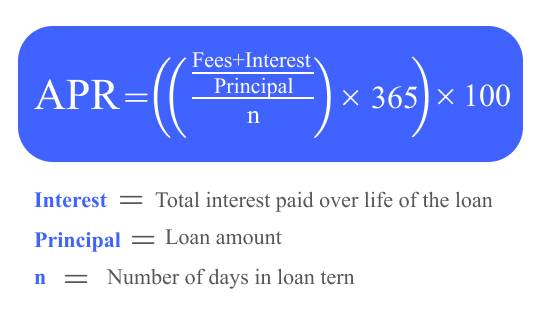# APY vs APR in crypto

If you want to put your liquidity into a pair and farm or stake a certain asset, you will be faced with the concept of annual profit. This is a kind of opportunity for you, as a crypto investor, to compare returns between assets or platforms, and calculate whether your returns are compounded and over what period of time. So, let’s figure out what APY means, what APR stands for, and the main difference between APY and APR.

11 min.
09.05.2023
24## What is APY in crypto

### What is APY?

To begin with, let’s give a description of the APY definition. APY stands for Annual Percentage Yield. APY refers to the annual rate of return on an investment, including compound interest that accumulates or grows with the balance. Compound interest is the interest accrued on the initial deposit + interest accrued on that interest. APY is accrual aware and therefore almost always higher than the stated annual interest rate. APY is a useful tool for estimating the real return on investment or the real interest rate paid on a loan.

How does APY work? For example, suppose an account with a certain bank offers an annual interest rate of 5%. If a user deposits \$1,000 into his account, then in a year he will have \$1,050 in his account.

Let’s say the account mentioned above earns interest on a monthly basis. If the same user deposits \$1,000 into this account, they will have \$1,051.16 in a year. The difference between these two amounts may seem small, but over time, the accumulated profit will be quite significant.

Here's how your profits can increase every month for a year with an annual percentage yield.

Period (month)BalanceProfit
1\$1004.17  \$4.17
2\$1008.35\$4.18
3\$1012.55\$4.20
4\$1016.77\$4.22
5\$1021.01\$4.24
6\$1025.26  \$4.25
7\$1029.53\$4.27
8\$1033.82\$4.29
9\$1038.13\$4.31
10\$1042.46\$4.33
11\$1046.80\$4.34
12\$1051.16\$4.36

Most savings accounts in traditional financial institutions offer very low APY rates, with the highest being around 0.70% and the lowest around 0.06%. As a result, any interest income from savings account deposits is quite insignificant compared to the returns on stock market investments.

### How does APY work in crypto?

APY meaning in crypto is the same.

We are used to hearing about APY in traditional savings. However, with the growing popularity of cryptocurrency, this metric has become an integral part of it. In cryptocurrency savings programs,  APY works similarly.

Cryptocurrency investors can earn APY on crypto placing them in savings accounts, providing liquidity to liquidity pools through yield farming, or, of course, by staking. This figure shows what percentage of profit you will receive per year by investing in farming or staking any pair of assets, taking into account constant reinvestment. For example, you start staking with an APR of 200% per annum. And reinvest your daily small profit in this staking. Thus, in a year you will receive a little more crypto with APY - about 280% (conditional numbers).

Percentage yield helps explain how much you can earn from your assets. In most cases, you will receive interest from the same cryptocurrency in which the deposit was made, however, there may be exceptions.

### How to calculate APY?

Interesting in how to find APY? You can calculate it using a special formula. APY formula is commonly used in traditional finance, where nominal interest rates are basically the same over a long period of time. It includes the nominal interest rate and the number of compound interest periods. The definitions of these two variables are as follows:

• The nominal interest rate is the interest rate before inflation.
• The period of compound interest is the time period between the last time interest is calculated and the time when it starts to be calculated again. For example, monthly compound interest means that interest will be calculated monthly. The period can be daily, monthly, annually, or any other period.

The percentage yield formula is the following:where:

i = Nominal interest rate

n = Number of periods

APYs in the cryptocurrency world are constantly changing. As a result, the APYs listed on staking and liquidity pools, cryptocurrency exchanges, are often only estimated. Volatility arises from changes in supply and demand for specific crypto assets. If the demand for a particular cryptocurrency asset increases, the interest rate and APY will usually increase as well.

The blockchain protocol used by projects also plays a role in the calculation of annual percentage yield, since the interest period may be different for each project. For example, some projects charge interest based on the number of blocks mined in one cycle, while others charge interest based on the time frame chosen. The longer the interest period, the higher the APY crypto will be. However, the difference is relatively minimal.

### Which crypto has the highest APY?

It is impossible to determine which crypto has the highest APY because it does not actually exist.

Annual percentage yield often depends on the cryptocurrency interest platform you are depositing on - they all offer their own, better or worse, DeFi interest rates. There are a number of key factors to check when looking for the best cryptocurrency interest accounts on the market. It is not only the safety of your funds but also what coins and yields are offered. But sometimes a high percentage is associated with unreliable projects.

## What is APR in crypto

### What is APR?

Now let's move on to the APR definition. APR stands for Annual Percentage Rate and means the simple interest rate that banks charge you for an entire year. It can be identical to annual percentage yield because they represent both interest and income. However, APR does not account for compound interest.

APR is often used to discuss terms for borrowers in traditional financing. For instance, the interest rate on a credit card that the borrower must pay. This interest rate can also refer to the interest paid to investors. Typically, the APY on loans is higher than the corresponding APR due to the compounding effect.

### What is an APR vs interest rate?

Some people are wondering: Is APR the same as an interest rate? But do not should to confuse interest rate vs APR. When it comes to loans, they have different meanings. The interest rate is the cost of borrowing the principal amount. The annual percentage rate (APR) is almost always higher than the interest rate, including other costs associated with borrowing money. That is, you will use the interest rate to calculate the monthly payment and use the APR to evaluate the entire cost of the loan and compare offers.

### APR vs EAR: what is the difference?

As we already know, APR means the nominal annual interest rate and EAR stands for "effective" percentage rate or effective APR. These are descriptions of the annual interest rate, not the monthly rate calculated on a loan or deposit. In general, APR is a simple interest rate per year, while EAR is a compound interest rate plus commission calculated per year. The EAR is often referred to as the "mathematically correct" interest rate for each year.

### What is APR in crypto staking?

The annual percentage rate shows exactly how much profit you can earn in a year by investing in this or that farming or staking. That is, if you see a pair of assets with an APR of 200%, it means that in 1 year you will receive 200% more funds from your deposit. Another case: you invest 100 tokens at 50% APR - in a year you get 150 tokens, of which 100 are your initial investment, and 50 tokens of profit.

The annual percentage rate in cryptocurrencies is the monetary value or reward that investors can receive by making their crypto tokens available for loans, taking interest rates into account. Several platforms encourage clients to deposit their crypto assets by offering them a high APR. The annual interest rate does not include compound interest.

### How to calculate APR?

To calculate APR it is also used special formula. The annual interest rate formula is the following:

APR = [(Fees + Interest) ÷ Principal] ÷ n × 365 × 100

Where:

Principal = Loan amount

N = number of days in the term### How does APR work?

For loans, the APR  includes the interest rate plus any fees charged by the lender, such as issuance, legal, or underwriting fees. There are two types of loans offered by exchanges:

1. Fixed lending protects your money for a certain period of time at a fixed rate (typically from seven to ninety days). The advantage of not touching your cryptocurrency is that it brings a higher interest rate. Flexible lending works similarly to a savings account. However, with flexible lending, you have the opportunity to withdraw your cryptocurrency at any time.

### Can I convert APY to APR?

Yes, you can. To calculate APY using APR:

• Take the APR and divide it by the number of compound interest periods.
• Add 1 to the result.
• Increase the result by the number of compound interest periods.
• Subtract 1 from the result.

### What is a good APR for crypto?

It is difficult to calculate a good APR in cryptocurrency because it depends on cryptocurrency rates, your contribution, and the income that you ultimately want to receive. The coins bring the highest percentage - from 5% to 25% on most exchanges.

## APY vs APR in crypto

To understand the main difference between APR and APY in cryptocurrency, and to determine which is more profitable, let's summarize. In simple words, APR is the interest rate per year. That is, how much as a percentage of your contribution you will receive in a year if you do nothing with your account? And APY means some “progressive rate”, in which the income paid out at each time interval is added to the initial contribution. Interest begins to accrue on the amount of the deposit, which has become larger, and with each payment, the amount of the deposit becomes higher. Therefore, APY for the year, as a result, is significantly higher.

Thus, banks promote their APY rather than APR. Also, it usually seems like a good investment for the client because APY is bigger than APR.

### How APY and APR are calculated?

The percentage of APY and APR depends on the TVL (blocked funds) in a particular pool. That is if there are no funds in the staking pool yet, and you are the first to deposit \$100 there, you will receive the entire daily reward of tokens that was provided by the project. If another person has invested \$100, the reward will already be divided among you, and you will receive only half. Therefore, with the growth of TVL and the popularity of the pair, the percentages of APY and APR will decrease.

### The nuances of annual interest

The problem is that APR and APY are far from the most accurate profit percentages. It comes down to 2 important factors:

1) Interest in staking and farming will definitely constantly change throughout the year due to various factors: TVL, decisions of the platform management, and the cost of the token in which interest is accrued.

If, for example, your pair had a small percentage of profit, then this will not affect your deposit in the same way. Up to 50% per annum usually does not change much. Maximum +-10–20%. But highly volatile assets with an initial promised APY of 1000% can fall by 100% daily and eventually fall to almost 0.

2) The second problem is the cost of the tokens themselves. If an investor stakes an unpopular and volatile token “X”, then he will receive annual profit in the same token. It must be remembered and kept in mind that over the year the price of the “X” token may fall, which means that your profit will be lower than the percentages originally declared by APR and APY. The more unreliable and highly volatile asset, the greater the risk of burning out on it, even despite the huge interest.

### APR vs APY: main differences

Here's what you need to know when comparing APR to APY:

APR APY
Interest rate plus fees, which are shown as an annual rateAnnual interest rate including the effect of interest accrual
Usually refers to the money you borrowUsually refers to the money you earn from investments
Lower annual interest helps you save money and is based on your creditA higher APY helps you make more money
Different types of APR depending on the financial productDoes not include fees

## The Bottom line

Every investor needs a method to compare investment opportunities and calculate profits. Both APY and APR are standard rates of return calculation used in both traditional finance and cryptocurrencies.

Today, we have told you all you should know about APY vs APR and how they work in crypto. We have explained to you what is an APY and what it means in crypto, as well as APR meaning, and how is APR calculated, and gave many other answers to your frequent questions.